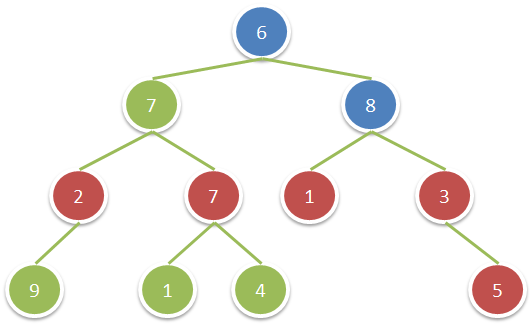Formatted question description: https://leetcode.ca/all/1315.html

# 1315. Sum of Nodes with Even-Valued Grandparent (Medium)

Given a binary tree, return the sum of values of nodes with even-valued grandparent.  (A grandparent of a node is the parent of its parent, if it exists.)

If there are no nodes with an even-valued grandparent, return 0.

Example 1:Input: root = [6,7,8,2,7,1,3,9,null,1,4,null,null,null,5]
Output: 18
Explanation: The red nodes are the nodes with even-value grandparent while the blue nodes are the even-value grandparents.


Constraints:

• The number of nodes in the tree is between 1 and 10^4.
• The value of nodes is between 1 and 100.

Related Topics:
Tree, Depth-first Search

## Solution 1. Pre-order Traversal

// OJ: https://leetcode.com/problems/sum-of-nodes-with-even-valued-grandparent/

// Time: O(N)
// Space: O(N)
class Solution {
int ans = 0;
vector<TreeNode*> v;
void preorder(TreeNode *root, int level) {
if (!root) return;
if (v.size() <= level) v.push_back(root);
else v[level] = root;
if (level - 2 >= 0 && v[level - 2]->val % 2 == 0) ans += root->val;
preorder(root->left, level + 1);
preorder(root->right, level + 1);
}
public:
int sumEvenGrandparent(TreeNode* root) {
preorder(root, 0);
return ans;
}
};


Java

/**
* Definition for a binary tree node.
* public class TreeNode {
*     int val;
*     TreeNode left;
*     TreeNode right;
*     TreeNode(int x) { val = x; }
* }
*/
class Solution {
public int sumEvenGrandparent(TreeNode root) {
int sum = 0;
nodeQueue.offer(root);
booleanQueue.offer(false);
while (!nodeQueue.isEmpty()) {
TreeNode node = nodeQueue.poll();
boolean isEven = booleanQueue.poll();
boolean curEven = node.val % 2 == 0;
TreeNode left = node.left, right = node.right;
if (left != null) {
if (isEven)
sum += left.val;
nodeQueue.offer(left);
booleanQueue.offer(curEven);
}
if (right != null) {
if (isEven)
sum += right.val;
nodeQueue.offer(right);
booleanQueue.offer(curEven);
}
}
return sum;
}
}